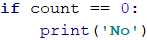# Chapter 8.1. Practical Exam Preparation - Part I

In the present chapter, we will examine a few problems with a level of difficulty that can be expected in the problems of the practical exam in “Programming Basics”. We will review and practice all the knowledge that was gained from this book and through the "Programming Basics" course.

## The "Programming Basics" Practical Exam

The Practical Exam contains 6 problems included, and you will have 4 hours to solve them. Each of the exam problems will cover one of the studied topics during the course. Problem topics are as follows:

• Problem with simple calculations (without conditions)
• Problem with simple condition
• Problem with more complex conditions
• Problem with a simple loop
• Problem with nested loops (drawing a figure on the console)
• Problem with nested loops and more complex logic

## The Online Evaluation System (Judge)

All exams and problems are automatically tested through the online Judge system: https://judge.softuni.org. For each of the problems, there are visible (zero points) tests that help you understand what is expected of the problem and fix your mistakes, as well as competition tests that are hidden and check if your solution is working properly.

How does the testing in the Judge system work? You upload the source code and from the menu below you choose to compile as a Python program. The program is being tested with a series of tests, giving points for each successful test.

## Problems with Simple Calculations

The first problem of the "Programming Basics" Practical Exam covers simple calculations without checks and loops. Here are a few examples:

### Problem: Triangle Area

 Triangle in the plain is defined by the coordinates of its three vertices. First, the vertex (x1, y1) is set. Then the other two vertices are set: (x2, y2) and (x3, y3) which lie on a common horizontal line i.e. they have the same Y coordinates). Write a program that calculates the triangle area by the coordinates of its three vertices.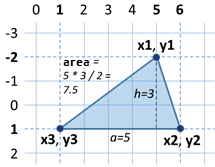#### Input Data

From the console 6 integers are read (one per line): x1, y1, x2, y2, x3, y3.

• All input numbers are in the range [-1000 … 1000].
• It's guaranteed that y2 = y3.

#### Output Data

Print on the console the triangle area.

#### Sample Input and Output

5
-2
6
1
1
1
7.5The side of the triangle a = 6 - 1 = 5
The height of the triangle h = 1 - (-2) = 3
The area of the triangle S = a * h / 2 = 5 * 3 / 2 = 7.5
4
1
-1
-3
3
-3
8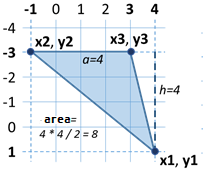The side of the triangle a = 3 - (-1) = 4
The height of the triangle h = 1 - (-3) = 4
The area of the triangle S = a * h / 2 = 4 * 4 / 2 = 8

#### Hints and Guidelines

It is extremely important in these types of tasks, in which some coordinates are given, to pay attention to the order in which they are submitted, as well as to correctly understand which of the coordinates we will use and in what way. In this case, the input is in order x1, y1, x2, y2, x3, y3. If we do not follow this sequence, the solution becomes wrong. First, we write the code that reads the input data: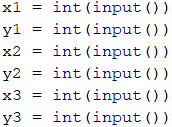We have to calculate the side and the height of the triangle. From the examples and the condition y2 = y3 we notice that one side is always parallel to the horizontal axis. It means that its length is equal to the length of the segment between its coordinates x2 and x3, which is equal to the difference between the larger and the smaller coordinates. Similarly, we can calculate the height. It will always be equal to the difference between y1 and y2(or y3, as they are equal). Since we do not know if x2 is greater than x3, or y1 will be below or above the triangle side, we will use the absolute values of the difference to always get positive numbers because one segment cannot have a negative length.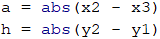We will use the formula familiar to us from school for finding the area of a triangle to calculate the area.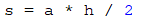The only thing left is to print the area on the console.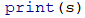### Problem: Bricks

Construction workers have to transfer a total of x bricks. The workers are w and work simultaneously. They transport the bricks in trolleys, each with a capacity of m bricks. Write a program that reads the integers x, w, and m, and calculates what is the minimum number of courses the workers need to do to transport the bricks.

#### Input Data

From the console 3 integers are read (one per line):

• The number of bricks x is read from the first line.
• The number of workers w is read from the second line.
• The capacity of the trolley m is read from the third line.

All input numbers are integers in the range [1 … 1000].

#### Output Data

Print on the console the minimum number of courses needed to transport the bricks.

#### Sample Input and Output

120
2
30
2 We have 2 workers, each transporting 30 bricks per course. In total, workers are transporting 60 bricks per course. To transport 120 bricks, exactly 2 courses are needed.
355
3
10
12 We have 3 workers, each transporting 10 bricks per course. In total, workers are transporting 30 bricks per course. To transport 355 bricks, exactly 12 courses are needed: 11 complete courses carry 330 bricks and the last 12th course carries the last 25 bricks.
5
12
30
1 We have 5 workers, each transporting 30 bricks per course. In total, workers are transporting 150 bricks per course. To transport 5 bricks, only 1 course is enough (although incomplete, with only 5 bricks).

#### Hints and Guidelines

The input is standard, and we only have to pay attention to the sequence in which we read the data: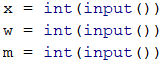We calculate how many bricks the workers transport in a single course: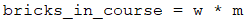By dividing the total number of bricks transported for 1 course, we will obtain the number of courses needed to carry them. We will use the math.ceil (…) function to round the result up. Remember to add import math at the beginning of the working file so that the math.ceil (…) function can work. When the bricks can be transferred with an exact number of courses, the division will return a whole number and there will be nothing to round. Accordingly, if not, the result of the division will be the number of exact courses but with a decimal fraction. The decimal part will be rounded up and we will get the required 1 course for the remaining bricks.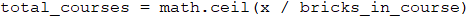In the end, we print the result on the console: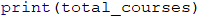## Problems with Simple Conditions

The second problem of the "Programming Basics" Practical Exam covers conditional statements and simple calculations. Here are a few examples:

### Problem: Point on Segment

On a horizontal line, a horizontal segment is placed, set with the x coordinates at both ends: first and second. A point is located on the same horizontal line and is set with its x coordinate. Write a program that checks whether the point is inside or outside of the segment and calculates the distance to the nearest end of the segment.

#### Input Data

From the console 3 integers are read (one per line):

• On the first line is the number first - one end of the segment.
• On the second line is the number second - the other end of the segment.
• On the third line is the number point - the location of the point.

All inputs are integers in the range [-1000 … 1000].

#### Output Data

Print the result on the console:

• On the first line, print "in" or "out" – whether the point is inside or outside the segment.
• On the second line, print the distance from the point to the nearest end of the segment.

#### Sample Input and Output

Input Output Visualization
10
5
7
in
2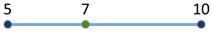Input Output Visualization
8
10
5
out
3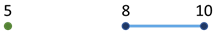Input Output Visualization
1
-2
3
out
2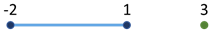#### Hints and Guidelines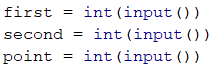Since we do not know which point is on the left and which is on the right, we will create two variables to mark this. Since the left point is always the one with the smaller x coordinate, we will use the min(…) function to find it. Accordingly, the right point is always the one with a larger x coordinate and we will use the max(…) function. We will also find the distance from the point x to the two points. Since we do not know their position relative to each other, we will use the abs(…) function to get a positive result: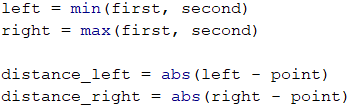The shorter of the two distances we will find by using min(…):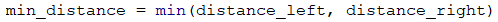What remains is to find whether the point is on or out of the line. The point will be on the line always when it matches one of the other two points or its x coordinate lies between them. Otherwise, the point is outside the line. After checking, we display one of the two messages ("in" or "out"), depending on which condition is satisfied: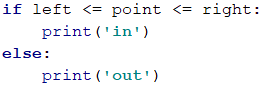Finally, we print the distance found before.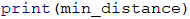### Problem: Point in Figure

Write a program that checks if a point (with coordinates x and y) is inside or outside of the given figure: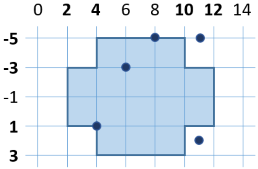#### Input Data

From the console are read two integers (one per line): x and y.

All inputs are integers in the range [-1000 … 1000].

#### Output Data

Print on the console "in" or "out" – whether the point is inside or outside the figure (the outline is inside).

#### Sample Input and Output

Input Output Input Output
8
-5
in 6
-3
in
Input Output Input Output
11
-5
out 11
2
out

#### Hints and Guidelines

To find out if the point is inside of the figure, we will divide the figure into 2 rectangles: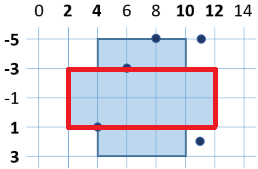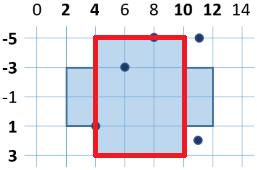A sufficient condition is the point to be located in one of them and be in the figure.

We read the input from the console: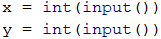We will initialize two variables that will mark whether the point is in one of the rectangles: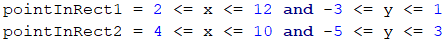When printing the message, we will check whether any of the variables has accepted a value of True. It's enough only one of them to be True so that the point is in the figure.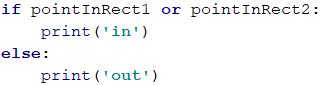## Problems with Complex Conditions

The third problem of the "Programming Basics" Practical Exam includes several nested checks combined with simple calculations. Here are a few examples:

### Problem: Date After 5 days

There are two numbers d (day) and m (month) that form a date. Write a program that prints the date that will be after 5 days. For example, 5 days after 28.03 is the date 2.04. We assume that the months: April, June, September, and November have 30 days, February has 28 days, and the rest have 31 days. Months to be printed with leading zero when they are single-digit numbers (e.g. 01, 08).

#### Input Data

The input is read from the console and consists of two lines:

• On the first line, there is an integer d in the interval [1… 31] - day. The number of the day does not exceed the number of days in the respective month (e.g. 28 for February).
• On the second line, there is an integer m in the interval [1… 12] - month. Month 1 is January, month 2 is February,…, month 12 is December. The month may contain a leading zero (e.g. April may be written as 4 or 04).

#### Output Data

Print a single line containing the date after 5 days in the format day.month on the console. The month must be a two-digit number with a leading zero, if necessary. The day must be written without a leading zero.

#### Sample Input and Output

Input Output Input Output
28
03
2.04 27
12
1.01
Input Output Input Output
25
1
30.01 26
02
3.03

#### Hints and Guidelines

We read the input data from the console: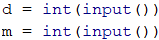To make our checks easier, we will create a variable that will contain the number of days that we have in the month we set: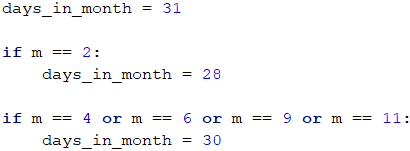We increase the day by 5: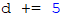We check if the day has exceeded the number of days in the month. If so, we must deduct the days of the month from the obtained day to calculate which day of the next month our day corresponds to: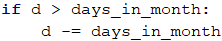After we have passed to the next month, this should be noted by increasing the initial one by 1. We need to check if it has become greater than 12 and if it has, to adjust it. Because we cannot skip more than one month when we increase by 5 days, the following check is enough: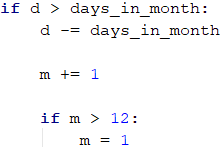The only thing that remains is to print the result on the console. It is important to format the output correctly to display the leading zero in the first 9 months. This is done by adding a formatted string %02d for the second element: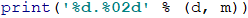### Problem: Sums 3 Numbers

There are 3 integers given. Write a program that checks if the sum of two of the numbers is equal to the third one. For example, if the numbers are 3, 5, and 2, the sum of two of the numbers is equal to the third one: 2 + 3 = 5.

#### Input Data

From the console are read three integers, one per line. The numbers are in the range [1 … 1000].

#### Output Data

• Print a single line on the console containing the solution of the problem in the format "a + b = c", where a, b and c are among the three input numbers and a ≤ b.
• If the problem has no solution, print “No” on the console.

#### Sample Input and Output

Input Output Input Output
3
5
2
2 + 3 = 5 2
2
4
2 + 2 = 4
Input Output Input Output
1
1
5
No 2
6
3
No

#### Hints and Guidelines

We take the input from the console: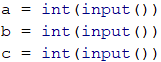We have to check if the sum of a pair of numbers is equal to the third number. We have three possible cases:

• a + b = c
• a + c = b
• b + c = a

We will write a template, which will later be complemented by the required code. If none of the above three conditions is met, we will make our program print "No":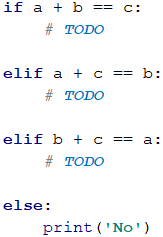Now it remains to understand the order in which the two addends will be displayed at the output of the program. For this purpose, we will make a nested condition, which checks which of the two numbers is the greater. In the first case, it will be as follows: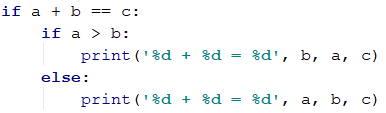Similarly, we will supplement the other two cases. The full code of the program will look like this: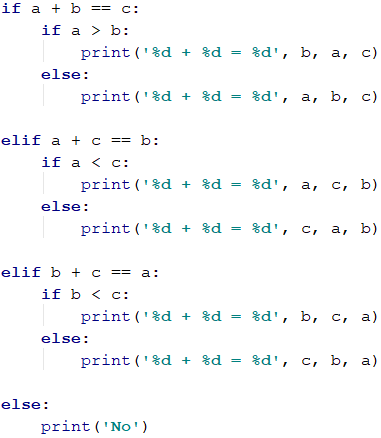## Problems with Simple Loops

The fourth problem of the "Programming Basics" Practical Exam includes a simple loop with simple logic in it. Here are a few examples:

### Problem: Sums Step 3

There are given n integers a1, a2, …, an. Calculate the sums:

• sum1 = a1 + a4 + a7 + … (the numbers are summed, starting from the first one with step of 3).
• sum2 = a2 + a5 + a8 + … (the numbers are summed, starting from the second one with step of 3).
• sum3 = a3 + a6 + a9 + … (the numbers are summed, starting from the third one with step of 3).

#### Input Data

The input data is read from the console. The first line contains an integer n (0 ≤ n ≤ 1000). The next n lines contain n integers in the interval [-1000 … 1000]: a1, a2, …, an.

#### Output Data

On the console, we should print 3 lines containing the 3 sums in a format such as in the example.

#### Sample Input and Output

Input Output Input Output Input Output
2
3
5
sum1 = 3
sum2 = 5
sum3 = 0
4
7
-2
6
12
sum1 = 19
sum2 = -2
sum3 = 6
5
3
5
2
7
8
sum1 = 10
sum2 = 13
sum3 = 2

#### Hints and Guidelines

We will take the count of numbers from the console and will declare starting values of the three sums.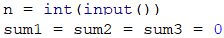Since we do not know in advance how many numbers we will process, we will take them one at a time in a loop which will be repeated n times and we will process them in the body of the loop.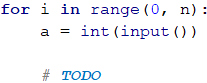To find out which of the three sums we need to add the number, we will divide its sequence number into three and we will use the remainder. We'll use the variable i which tracks the number of runs of the loop, to find out which sequence number we are at. When the remainder of i/3 is zero, it means we will add this number to the first sum, when it's 1 to the second, and when it's 2 to the third: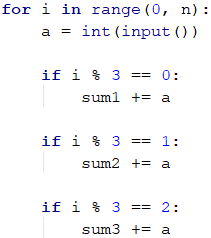Finally, we will print the result on the console in the required format: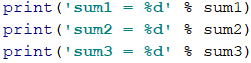### Problem: Increasing Elements

A series of n numbers is given: a1, a2, , an. Calculate the length of the longest increasing sequence of consecutive elements in the series of numbers.

#### Input Data

The input data is read from the console. The first line contains an integer n (0 ≤ n ≤ 1000). The next n lines contain n integers in the interval [-1000 … 1000]: a1, a2, , an.

#### Output Data

Print on the console one number – the length of the longest increasing sequence.

#### Sample Input and Output

Input Output Input Output Input Output Input Output
3
5
2
4
2 4
2
8
7
6
2 4
1
2
4
4
3 4
5
6
7
8
4

#### Hints and Guidelines

To solve this problem, we need to think in a bit more algorithmic way. A sequence of numbers is given to us, and we need to check whether each subsequent one will be larger than the previous one and if so, we count how long is the sequence in which this condition is fulfilled. Then we have to find which sequence of these is the longest one. To do this, let's create some variables that we will use during solving the problem: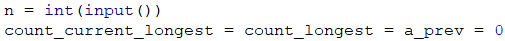The variable n is the count of numbers we get from the console. In count_current_longest we'll keep the number of elements in the increasing sequence we are currently counting. For example, in the sequence: 5, 6, 1, 2, 3 count_current_longest will be 2 when we reach the second element of the counting (5, 6, 1, 2, 3) and will become 3 when we have reached the last element (5, 6, 1, 2, 3) because the increasing row 1, 2, 3 has 3 elements. We will use count_longest to keep the longest increasing sequence. The other variables we will use are a - the number we are at at the moment, and a_prev - the previous number, which we will compare with a to find out whether the row is growing.

We begin to run the numbers and check if the present number a is larger than the previous a_prev. If this is true, then the row is growing, and we need to increase its number by 1. This is stored in the variable that tracks the length of the sequence we are currently in – count_current_longest. If the number a isn't bigger than the previous one, it means that a new sequence starts, and we have to start the count from 1. Finally, after all the checks are done, a_prev becomes the number that we're currently using, and we start the loop from the beginning with the next entered a.

Here is a sample implementation of the algorithm described: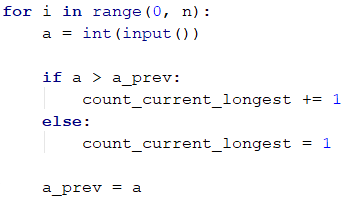What remains is to see which of all sequences is the longest. We will do this by checking in the loop if the sequence we are currently in has become longer than the longest one by now. The whole loop will look like this: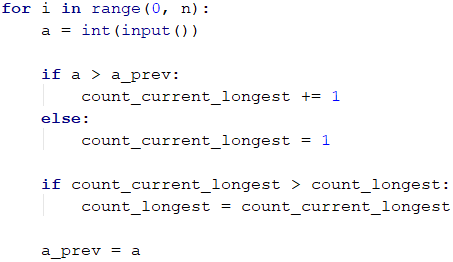Finally, we print the length of the longest sequence found: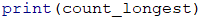## Problems for Drawing Figures on The Console

The fifth problem of the "Programming Basics" Practical Exam requires using one or several nested loops for drawing a figure on the console. Logical reasoning, simple calculations, and conditional statements may be required. The problem tests the ability of students to think logically and invent simple algorithms for solving problems, i.e. to think algorithmically. Here are some examples of Exam Problems:

### Problem: Perfect Diamond

Write a function that takes as a parameter n and draws a perfect diamond with size n as in the examples below.

#### Input Data

The input is an integer n in the interval [1… 1000].

#### Output Data

The diamond should be printed on the console as in the examples below.

#### Sample Input and Output

Input Output Input Output
2  *
*-*
 *
3   *
 *-*
*-*-*
 *-*
  *
Input Output Input Output
4    *
  *-*
 *-*-*
*-*-*-*
 *-*-*
  *-*
   *
5     *
   *-*
  *-*-*
 *-*-*-*
*-*-*-*-*
 *-*-*-*
  *-*-*
   *-*
    *

#### Hints and Guidelines

In tasks for drawing figures, the most important thing to consider is the sequence in which we will draw. Which elements are repeated and with what steps? We can see that the top and the bottom parts of the diamond are the same. The easiest way to solve the problem is by creating a loop that draws the upper part, and then another loop that draws the bottom part (opposite to the top one).

First, we read the number n from the console: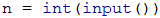We start drawing the upper half of the diamond. We see that each line starts with some whitespaces and *. If we take a closer look, we will notice that the whitespaces are always equal to n - index of row - 1 (the first row is n-1, the second – n-2, etc.). We will start by drawing the number of whitespaces and the first asterisk. Let's not forget to use print(…, end='') instead of just print(…) to stay on the same line. At the end of the line, we write print () to move to a new line. Notice that we start counting from 1, not 0. Then all we have to do is add -* a few times to complete the line.

Here is the complete code for drawing the upper part of the diamond: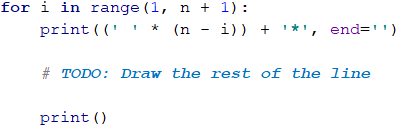What remains is to complete each line with the required number of -* elements. On each row we have to add i - 1 such items (on the first 1-1 -> 0, the second -> 1, etc.)

Here is the complete code for drawing the upper part of the diamond: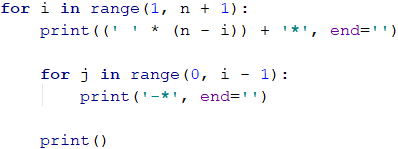To draw the bottom part of the diamond, we have to reverse the upper part. We'll count from n - 2 because if we start from n - 1, we will draw the middle row twice. Be sure to add reversed (…) to range (…) of the main loop.

Here is the code for drawing the bottom part of the diamond: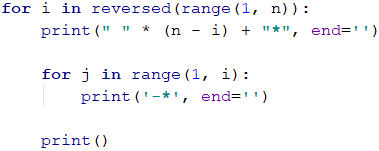What remains is to assemble the whole program by first reading the input, printing the top part of the diamond, and then the bottom part of the diamond.

### Problem: Rectangle with Stars

Write a function that takes as a parameter an integer n and draws a rectangle with size n with 2 asterisks in the center as in the examples below.

#### Input Data

The parameter is an integer n in the range [2 … 1000].

#### Output Data

The rectangle should be printed on the console as in the examples below.

#### Sample Input and Output

Input Output Input Output
2 %%%%
%**%
%%%%
3 %%%%%%
%    %
% ** %
%    %
%%%%%%
Input Output Input Output
4 %%%%%%%%
%      %
%  **  %
%      %
%%%%%%%%
5 %%%%%%%%%%
%        %
%        %
%   **   %
%        %
%        %
%%%%%%%%%%

#### Hints and Guidelines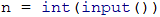The first thing we can easily notice is that the first and the last rows contain 2 * n symbols %. We will start with this and then draw the middle part of the rectangle: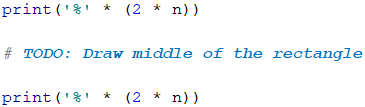From the examples, we see that the middle part of the figure always has an odd number of rows. Note that when an even number is set, the number of rows is equal to the previous odd number (2 -> 1, 4 -> 3, etc.). We create a variable that represents the number of rows that our rectangle will have, and correct it if the number n is even. Then we will draw a rectangle without the asterisks. Each row has for the beginning and at the end the symbol % and between them 2 * n - 2 whitespaces (the width is 2 * n and we subtract 2 for the two percent at the end). Do not forget to move the code or the last line after the loop: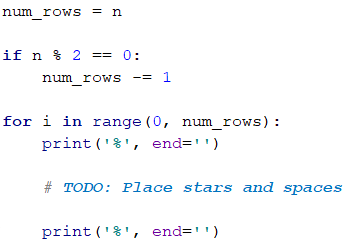We can start and test the code so far. Everything without the two asterisks in the middle should work correctly.

Now, in the body of the loop let's add the asterisks. We'll check if we're on the middle row. If we are in the middle, (we will check this by comparing i with floor(num_rows / 2)), we will draw the row together with the asterisks, if not – we will draw a normal row. Remember to add from the math import floor at the beginning of the work file so that we can use the floor(…) function. The line with the asterisks has n-2 whitespaces (n is half the length and we remove the asterisk and the percentage), two asterisks, and again n-2 whitespaces. We leave out of the check the two percent at the beginning and the end of the row: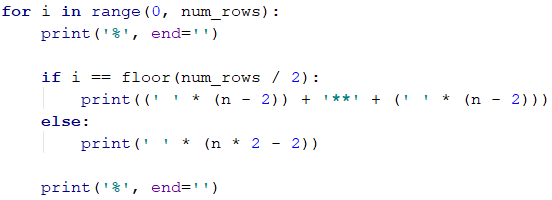## Problems with Nested Loops

The last (sixth) problem of the "Programming Basics" Practical Exam requires using of several nested loops and more complex logic inside them. The problems examine participants' ability to think algorithmically and to solve non-trivial coding problems that require nested loops. Here are some examples of exam problems.

### Problem: Increasing 4 Numbers

For a given pair of numbers, a and b generate all four numbers n1, n2, n3, n4, for which a ≤ n1 < n2 < n3 < n4 ≤ b.

#### Input Data

The input contains two integers a and b in the interval [0… 1000], one per line.

#### Output Data

The output contains all numbers in batches of four, in ascending order, one per line.

#### Sample Input and Output

Input Output Input Output
3
7
3 4 5 6
3 4 5 7
3 4 6 7
3 5 6 7
4 5 6 7
15
20
15 16 17 18
15 16 17 19
15 16 17 20
15 16 18 19
15 16 18 20
15 16 19 20
15 17 18 19
15 17 18 20
15 17 19 20
15 18 19 20
16 17 18 19
16 17 18 20
16 17 19 20
16 18 19 20
17 18 19 20
Input Output Input Output
5
7
No 10
13
10 11 12 13

#### Hints and Guidelines

We read the input data from the console. We also create the additional variable count, which will keep track of whether an existing number of ranges: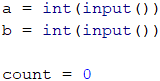We will most easily solve the problem if we logically divide it into parts. If we are required to draw all the rows from a number between a and b, we will do it using one loop that takes all the numbers from a to b. Let's think about how to do this with a series of two numbers. The answer is easy – we will use nested loops: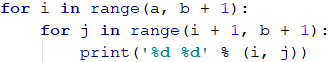We can test the incomplete program to see if it's accurate so far. It must print all pairs of numbers i, j for which i ≤ j.

Since each next number of the row must be greater than the previous one, the second loop will run around i + 1 (the next greater number). Accordingly, if there is no sequence of two incremental numbers (a and b are equal), the second loop will not be fulfilled, and nothing will be printed on the console.

Similarly, what remains is to implement the nested loops for four numbers. We will add an increase of the counter (count) that we initialized to know if there is such a sequence: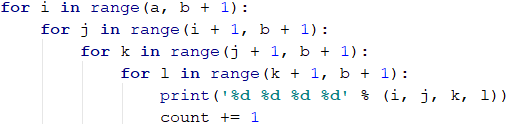Finally, we will check if the counter is equal to 0 and we will print "No" on the console accordingly, if so.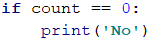### Problem: Generating Rectangles

By a given number n and a minimum area m, generate all possible rectangles with integer coordinates in the range [-n … n] with an area of at least m. The generated rectangles must be printed in the following format:

(left, top) (right, bottom) -> area

Rectangles are defined using the top left and bottom right corners. The following inequalities are in effect:

• -n ≤ left < right ≤ n
• -n ≤ top < bottom ≤ n

#### Input Data

Two numbers are entered from the console, one per line:

• Integer n in the range [1 … 100] - sets the minimum and maximum coordinate of a vertex.
• Integer m in the interval [0 … 50 000] - sets the minimum area of the generated rectangles.

#### Output Data

• The described rectangles should be printed on the console in a format such as in the examples below.
• If there are no rectangles for the specified n and m, then print "No".
• The order of rectangles in the output is not important.

#### Sample Input and Output

Input Output Input Output
1
2
(-1, -1) (0, 1) -> 2
(-1, -1) (1, 0) -> 2
(-1, -1) (1, 1) -> 4
(-1, 0) (1, 1) -> 2
(0, -1) (1, 1) -> 2
2
17
No
Input Output
3
36
(-3, -3) (3, 3) -> 36

#### Hints and Guidelines

Let's read the input from the console. Again, we will create a counter in which we will store the number of rectangles found: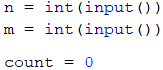It is very important to be able to imagine the problem before we begin to solve it. In our case, it is required to search for rectangles in a coordinate system. The thing we know is that the left point will always have the coordinate x, smaller than the right one. Accordingly, the upper one will always have a smaller y coordinate than the lower one. To find all the rectangles, we'll have to create a loop similar to the previous problem, but this time, not every next loop will start from the next number because some of the coordinates can be equal (for example left and top):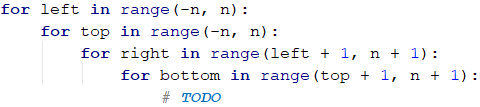With the variables left and right we will follow the coordinates along the horizontal, and with top and bottom - along the vertical. The important thing here is knowing the corresponding coordinates so we can correctly calculate the sides of the rectangle. Now we have to find the area of the rectangle and check if it is greater than or equal to m. One side will be the difference between left and right and the other one – between top and bottom. Since the coordinates may be eventually interchanged, we will use absolute values. Again, we add the counter in the loop, counting only the rectangles we write. It is important to note that the writing order is left, top, right, bottom, as it is set in the problem's description: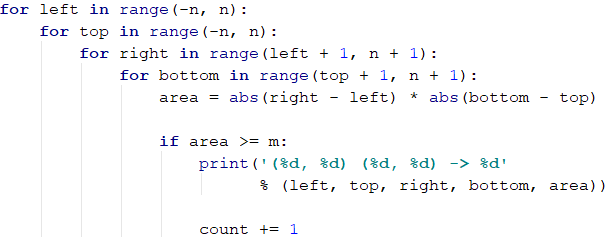Finally, we print “No”, if there are no such rectangles.